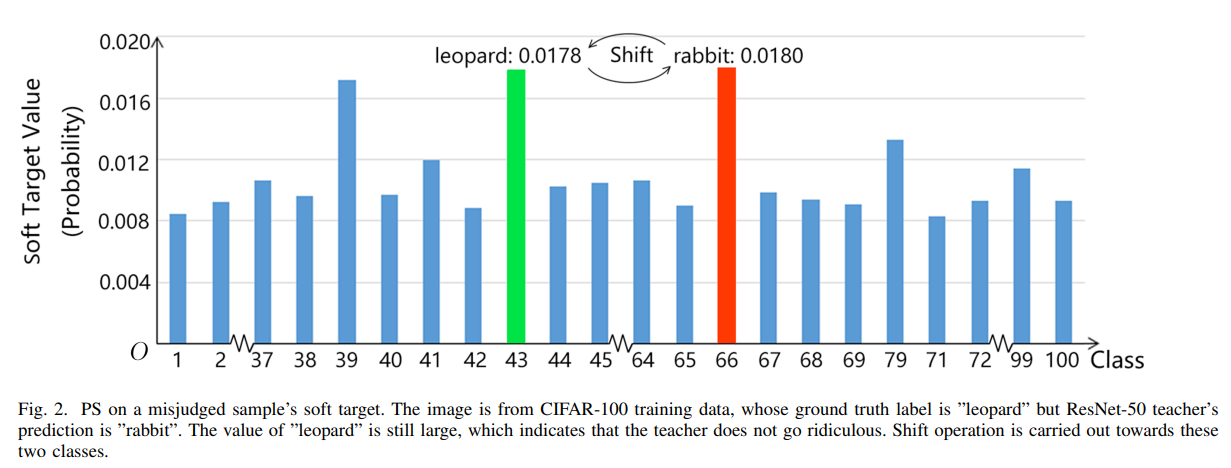# Probability Shift using KD_Lib¶

Paper

• Given an incorrect soft target, the probability shift algorithm simply swaps the value of ground truth (the theoretical maximum) and the value of predicted class (the predicted maximum), to assure the maximum confidence is reached at ground truth labelTo use the probability shift algorithm to train a student on MNIST for 5 epcohs -

import torch
import torch.nn as nn
import torch.optim as optim
from torchvision import datasets, transforms
from KD_Lib.KD import ProbShift

# Define datasets, dataloaders, models and optimizers

datasets.MNIST(
"mnist_data",
train=True,
transform=transforms.Compose(
[transforms.ToTensor(), transforms.Normalize((0.1307,), (0.3081,))]
),
),
batch_size=32,
shuffle=True,
)

datasets.MNIST(
"mnist_data",
train=False,
transform=transforms.Compose(
[transforms.ToTensor(), transforms.Normalize((0.1307,), (0.3081,))]
),
),
batch_size=32,
shuffle=True,
)

# Set device to be trained on

device = torch.device("cuda:0" if torch.cuda.is_available() else "cpu")

# Define student and teacher models

# Define optimizers

teacher_optimizer = optim.SGD(teacher_model.parameters(), lr=0.01)
student_optimizer = optim.SGD(student_model.parameters(), lr=0.01)

# Train using KD_Lib